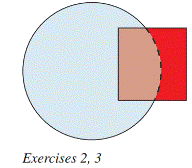Chapter 8.1, Problem 3EElementary Geometry For College St...

7th Edition
Alexander + 2 others
ISBN: 9781337614085

Solutions

Chapter
SectionElementary Geometry For College St...

7th Edition
Alexander + 2 others
ISBN: 9781337614085
Textbook Problem

Consider the information in Exercise 2, but suppose you know that the area of the region defined by the intersection of the square and the circle measures 5. What is the area of the entire colored region?2. The area of the square is 12, and the area of the circle is 30. Does the area of the entire shaded region equal 42? Why or why not?To determine

To Find:

The area of the entire colored region.

Explanation

Calculation:

Given,

The area of the square =12 unit2

The area of the circle =30 unit2

From the figure,

The area of the circle =12

5+z=12

Add 5 on both sides,

5+z5=125

z=7(1)

The area of the square =30

5+y=30

Still sussing out bartleby?

Check out a sample textbook solution.

See a sample solution

The Solution to Your Study Problems

Bartleby provides explanations to thousands of textbook problems written by our experts, many with advanced degrees!

Get Started

In Exercises 516, evaluate the given quantity. log100,000

Finite Mathematics and Applied Calculus (MindTap Course List)

For

Study Guide for Stewart's Multivariable Calculus, 8th

What is the expected value of a random variable? Give an example.

Finite Mathematics for the Managerial, Life, and Social Sciences

With an initial estimate of −1, use Newton’s Method once to estimate a zero of f(x) = 5x2 + 15x + 9.

Study Guide for Stewart's Single Variable Calculus: Early Transcendentals, 8th